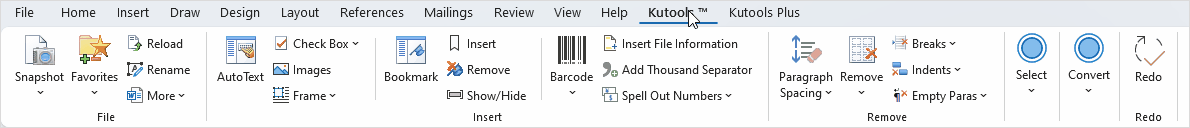## How to calculate days or hours between two dates or times In Word document?

Normally, we can calculate the number of days between two dates in Excel worksheet quickly and easily, but, have you ever tried to get the number of days between two given dates in a Word document?

Calculate difference between two dates in Word document with VBA code

Calculate difference between two times in Word document with VBA code

#### Calculate difference between two dates in Word document with VBA code

To calculate the number of days between two given dates, the below VBA code can do you a favor, please do as this:

1. Hold down the ALT + F11 keys to open the Microsoft Visual Basic for Applications window.

2. And then, click Insert > Module, copy and paste below code into the opened blank module:

VBA code: Calculate difference between two dates

``````Sub CalculateDateDifference()
Dim xStartDate As Date
Dim xEndDate As Date
Dim xDay As Long
On Error Resume Next
xStartDate = InputBox("Enter the start date", "KuTools for Word", "")
xEndDate = InputBox("Enter the end date", "KuTools for Word", "")
If (InStr(1, Str(xStartDate), ":") > 0) Or (InStr(1, Str(xEndDate), ":") > 0) Then
MsgBox "please input current date", vbInformation, "KuTools for Excel"
Exit Sub
End If
xDay = DateDiff("d", xStartDate, xEndDate)
MsgBox "There are " & xDay & " days left from " & xStartDate & " to " & xEndDate & vbCrLf, vbInformation, "KuTools for Word"
End Sub
``````

3. And then, press F5 key to run this code, in the consecutive dialog boxes, enter the start date and end date that you want to use, see screenshot: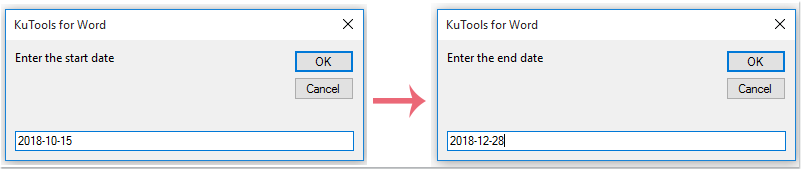4. Then, click OK button, and you will get the result that you want, see screenshot: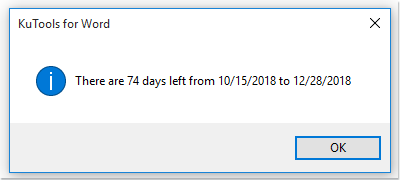#### Calculate difference between two times in Word document with VBA code

Here is another VBA code which can help you to calculate the difference between two given times, please do as this:

1. Hold down the ALT + F11 keys to open the Microsoft Visual Basic for Applications window.

2. And then, click Insert > Module, copy and paste below code into the opened blank module:

VBA code: Calculate difference between two times

``````Sub CalculateTimeDifference()
Dim xStartDate As Date
Dim xEndDate As Date
Dim xTime As Long
Dim xHour As Long
On Error Resume Next
xStartDate = InputBox("Enter the start time", "KuTools for Word", "")
xEndDate = InputBox("Enter the end time", "KuTools for Word", "")
Debug.Print Str(xStartDate)
If (Str(xStartDate) = " 0:00:00") Or (Str(xEndDate) = " 0:00:00") _
Or (Str(xStartDate) = " 12:00:00 AM") Or (Str(xEndDate) = " 12:00:00 AM") Then
MsgBox "please input the time", vbInformation, "KuTools for Excel"
Exit Sub
ElseIf xStartDate > xEndDate Then
MsgBox " The start time is not larger than the end time!", vbInformation, "KuTools for Excel"
Exit Sub
End If
xTime = DateDiff("s", xStartDate, xEndDate)
xHour = xTime \ 3600
xTime = xTime - xHour * 3600
MsgBox "There are " & xHour & " hours " & xTime \ 60 & " minutes " & xTime - (xTime \ 60) * 60 _
& " seconds left from " & xStartDate & " to " & xEndDate & vbCrLf, vbInformation, "KuTools for Word"
End Sub
``````

3. And then, press F5 key to run this code, in the consecutive dialog boxes, enter the start time and end time that you want to use to calculate difference, see screenshot: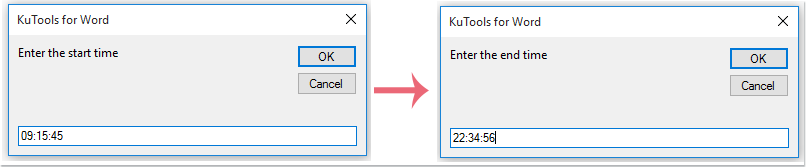4. Then, click OK button, and the time difference between two given times has been calculated and displayed as following screenshot shown: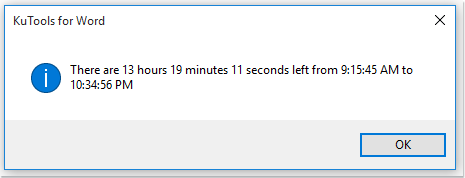### Best Office Productivity Tools

#### Kutools for Word - Elevate Your Word Experience with Over 100 Remarkable Features!

Dive into the highlighted features below or click here to explore the full power of Kutools for Word.

🔍 Precision Selections: Pinpoint specific pages  /  tables  /  shapes  /  heading paragraphs  /  Navigate with ease using our Select Group